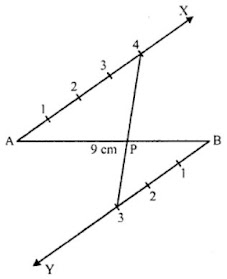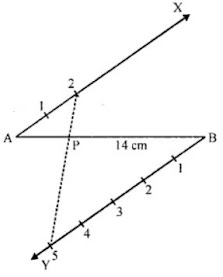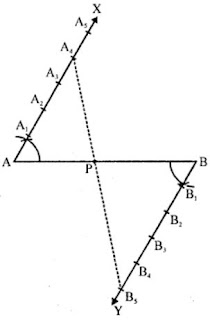# RD Sharma Solutions Chapter 11 Constructions Exercise 11.1 Class 10 Maths

 Chapter Name RD Sharma Chapter 11 Constructions Book Name RD Sharma Mathematics for Class 10 Other Exercises Exercise 11.1Exercise 11.2Exercise 11.3 Related Study NCERT Solutions for Class 10 Maths

### Exercise 11.1 Solutions

1. Determine a point which divides a line segment of length 12 cm internally in the ratio 2 : 3. Also justify your construction.

Solution

Steps of construction :

1. Draw a line segment AB = 12 cm.
2. Draw a ray AX at A making an acute angle with AB.
3. From B, draw another ray BY parallel to AX.
4. Cut off 2 equal parts from AX and 3 equal parts from BY.
5. Join 2 and 3 which intersects AB at P.
P is the required point which divides AB in the ratio of 2 : 3 internally.2. Divide a line segment of length 9 cm internally in the ratio 4 : 3. Also, give justification of the construction.

Solution

Steps of construction :

1. Draw a line segment AB = 9 cm.
2. Draw a ray AX making an acute angle with AB.
3. From B, draw another ray BY parallel to AX.
4. Cut off 4 equal parts from AX and 3 parts from BY.
5. Join 4 and 3 which intersects AB at P.
P is the required point which divides AB in the ratio of 4 : 3 internally.3. Divide a line segment of length 14 cm internally in the ratio 2 : 5. Also justify your construction.

Solution

Steps of construction :

1. Draw a line segment AB = 14 cm.
2. Draw a ray AX making an acute angle with AB.
3. From B, draw another ray BY parallel to AX.
4. From AX, cut off 2 equal parts and from B, cut off 5 equal parts.
5. Join 2 and 5 which intersects AB at P.
P is the required point which divides AB in the ratio of 2 : 5 internally.4. Draw a line segment of length 8 cm and divide it internally in the ratio 4 : 5.

Solution

Steps of construction :

1. Draw a line segment AB = 8 cm.
2. Draw a ray AX making an acute angle with ∠BAX = 60° with AB.
3. Draw a ray BY parallel to AX by making an acute angle ∠ABY = ∠BAX.
4. Mark four points A1, A2, A3, A4 on AX and five points B1, B2, B3, B4, Bs on BY in such a way that AA1 = A1A2 = A2A3 = A3A4 .
5. Join A4B5.
6. Let this line intersect AB at a point P.
Thus, P is the point dividing the line segment AB internally in the ratio of 4 : 5.